Courses

# Short & Long Answer Question: Work, Energy & Power JEE Notes | EduRev

## Class 11 : Short & Long Answer Question: Work, Energy & Power JEE Notes | EduRev

The document Short & Long Answer Question: Work, Energy & Power JEE Notes | EduRev is a part of the Class 11 Course Physics For JEE.
All you need of Class 11 at this link: Class 11

Q.1 (a). If a force acts perpendicular to the Action of motion of a body, what is the amount of  done? (b). A body is moving at constant speed over a frictionless surface. What is the work done by the weight?
Answer:
(a). The work done is zero because there is no Placement in the direction of force.

(b). Work done by the weight is zero, since the force and the displacement are at right angles to each other.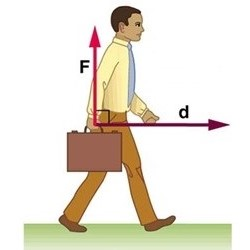Q.2 The earth moving around the sun in a circular orbit is acted upon by a force and hence work must be done on the earth by the force. Do you agree by this statement?
Answer:
The statement is wrong. The gravitational force is a conservative force. So the work done by the gravitational force over every complete orbit of the earth is zero.
Q.3 A body is moving along a circular path. How much work is done by the centripetal force?
Or
Why is the work done by centripetal force zero?

Answer: For a body moving along a circular path, the centripetal force acts along the radius while the displacement is tangential i.e., θ=90,, therefore W =Fscos 90=0.
Q.4 A body of mass m is moving in a circle of radius r with a constant speed v. The force on the body is mυ2/r and is directed towards the center. What is the work done by this force in moving the body over half the circumference of the circle?
Answer: Work done by the force is zero, because the direction of displacement of the body at every point is perpendicular to the direction of force acting on the body.
Q.5 Is it possible that a body be in accelerated motion under a force acting on the body, yet no work is being done by the force? Explain your answer giving a suitable example.
Answer:  Yes, it is possible, when the force is perpendicular to the direction of motion. The moon revolves round the earth under the centripetal force of attraction of the earth, but earth does no work on the moon.
Q.6 How much work is done by a coolie walking on a horizontal platform with a load on his head?

Answer: Zero. In order to balance the load on his head, the coolie applies a force on it in the upward direction equal to its weight. His displacement is along the horizontal direction. Thus the angle between force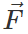and displacement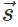is 90. Therefore work done, W=Fs cosθ =Fs cos90=0.
Q.7 A porter moving vertically up the stairs with a suitcase on his head does work. Why?
Answer:
The porter lifts the suitcase vertically to the upstairs. Force has to be applied on the suitcase against the force of gravitation. Hence the porter does work.
Q.8 Does the work done in raising a suitcase on to a platform depend upon how fast it is raised up?

Answer: No. The work done depends on the force of gravity and the height through which the suitcase is raised. It does not depend on the time rate with which the suitcase is raised.
Q.9 In a tug of war one team is slowly giving way to the other. What work is being done and by whom?

Answer: The work is done by the winning team and is equal to the product of resultant force applied by the two teams and displacement that the losing team suffers.

Q.10 A man rowing boat upstream is at rest with respect to the shore. Is he doing work?

Answer: The boat is at rest with respect to the shore but it is moving upstream with respect to water. The man is doing work relative to the stream because he is applying force to produce relative motion between the boat and the stream. But he does no work relative to the shore as displacement relative to the shore is zero.
Q.11 Mountain roads rarely go straight up but wind up gradually. Why?

Answer: If the roads go straight up, the angle of slope θ would be large. In that case frictional force (f= μ mgcosθ) would be less and the vehicles may slip. Moreover, while going up a large slope, a greater power would be required (as it will take less time).
Q.12 What sort of energy is associated with a bird flying in air?

Answer:  A flying bird possesses both potential and kinetic energies because it is at a certain height above the ground and moving with certain velocity.
Q.13 When an arrow is shot, where from the arrow will acquire its kinetic energy?

Answer: A stretched bow possesses potential energy on account of a change in its shape. To shoot an arrow, the bow is released. The potential energy of the bow is converted into the kinetic energy of the arrow.
Q.14 Can a body have energy without momentum?

Answer: Yes, there is an internal energy in a body due to the thermal agitation of the particles of the body, while the vector sum of the momenta of the moving particles may be zero.
Q.15 Can a body have momentum without energy?

Answer: No, if a body has momentum, it must be in motion and consequently possess kinetic energy.

Q.16 A light body and a heavy body have the same momentum. Which one will have greater kinetic energy? Or Two bodies of unequal masses have same linear momentum, which one has greater kinetic energy?
Answer:
Kinetic energy,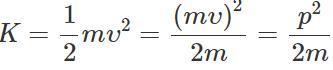For constant p, K∝ 1/m Thus the lighter body has more kinetic energy than the heavier body.
Q.17 A light body and a heavy body have the same kinetic energy. Which one will have the greater momentum?
Answer:
Kinetic energy,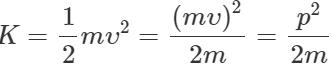or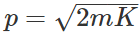i.e., p∝√m Thus the heavier body has a greater momentum than the lighter one.
Q.18 How does the kinetic energy of a body change if its momentum is doubled?
Answer: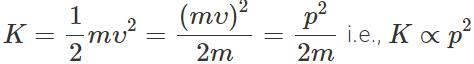When the momentum of a body is doubled, its kinetic energy becomes four times the initial kinetic energy.
Q.19 Two bodies of masses m1 and m2 have the same linear momentum. What is the ratio of their kinetic energies?

Answer: If υ1 and υ2 are the velocities of two bodies having masses m1 and respectively, then m1υ1=m2υ2  or m21υ21=m22υ22 or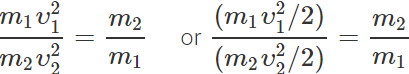or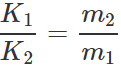Q.20 Two bodies of masses m1 and m2 have equal kinetic energies. What is the ratio of their linear momenta?
Answer: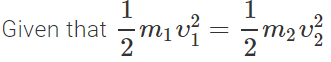or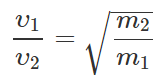or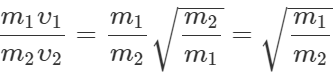or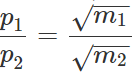Q.21 Can there be a situation in which E−U<0?

Answer: No. As E=K+U or K=E−U But kinetic energy K cannot be negative. So E−U is never less than zero.
Q.22 Can the overall energy of a body be negative?
Answer:
Yes. As E=K+U,when U is negative and has magnitude greater than that of K, E is negative. For example, the energy of an electron bound to the nucleus of an atom is negative.
Q.23 Does the potential energy of a spring decrease or increase when it is compressed or stretched?

Answer: The potential of the spring increases because work is done on it when it is compressed or stretched.
Q.24  If a block attached to a spring (whose other end is rigidly fixed) is pulled up to distance x0 and released, the amplitude of its motion cannot exceed ±x0. Why?

Answer: If the amplitude of motion exceeds ±x0,, then the potential energy would be greater than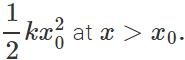So its K.E. =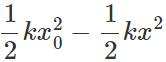would be negative. This is impossible. Hence the block cannot go beyond x=±x0.
Q.25 Springs A and B are identical except that A is stiffer than B, i.e., force constant kA>kB. In which spring is more work expended if they are stretched by the same amount?

Answer: Work done in stretching a spring of force constant k through a distance x,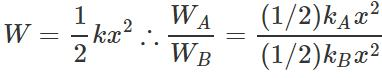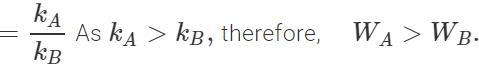Q.26 Springs A and B are identical except that A is stiffer than B. In which spring is more work expended if they are stretched by the same force?
Answer: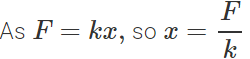For same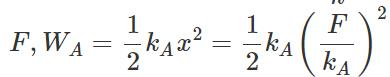and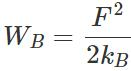∴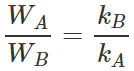As kA>kB  therefore, WA<WB.
Q.27 Which of the two: kilowatt hour or electron volt is a bigger unit of energy and by what factor?

Answer: Kilowatt hour (kWh) is a bigger unit of energy.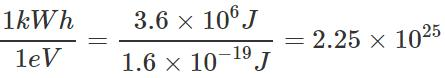Q.28 Will water at the foot of the fall be at a different temperature from that at the top? If yes, explain.

Answer: When water falls from a height, its P.E. is converted into kinetic energy and its velocity is maximum at the foot of the fall. The velocity is suddenly reduced to zero, and hence its kinetic energy gets converted into heat energy raising its temperature.
Q.29 Is it necessary that work done in the motion of a body over a closed loop is zero for every force in nature? Why?

Answer: No. The work done in the motion of body over a closed loop is zero only in case of a conservative force but it is not zero in case of a non-conservative force like friction.
Q.30 When a constant force is applied to a body moving with constant acceleration, is the power of the force constant? If not, how would force have to vary with speed for power to be constant?

Answer: Power, P=Fυ As the body is moving with acceleration, υ changes and so P also changes, F being constant. For P to be constant, Fυ=a constant or(F∝1/υ). Thus as υ increases, F should decrease to keep P constant.
Q.31 What are central forces? Are they conservative in nature?

Answer: Force between two objects is called a central force, if it acts between them along the line joining their centers. Electrostatic force between the charges and magnetic force between two poles are central forces and are conservative forces.
Q.32 When is the exchange of energy maximum during an elastic collision?

Answer: Energy exchange will be maximum if the two colliding bodies are of equal masses.
Q.33 Is whole of the kinetic energy lost in any perfectly inelastic collision?
Answer:
No, only that much amount of kinetic energy is lost as is necessary for the conservation of momentum.
Q.34 Can you associate potential energy with a non-conservative force?
Answer:
No, P.E. can be associated only with conservative force.
Q.35 Two bodies moving towards each other collide and move away in opposite directions. There is some rise of temperature of the bodies in the process. Explain the reason for the rise of temperature and state what type of collision is it.

Answer: Involved collision of the process is inelastic because the bodies suffer loss of kinetic energy which appears in the form of heat energy raising the temperature of the bodies.
Q.36 A spark is produced, when two stones are struck against each other. Why?
Answer:
The work done in striking the two stones against each other gets converted into heat. This appears as a spark.
Q.37 A lorry and a car with the same kinetic energy are brought to rest by the application of the brakes which provide equal retarding force. Which of them will come to rest in a shorter distance?

Answer: By work-energy theorem, Loss in K.E. of the vehicle =Work done against retarding force =Retarding force x distance travelled ∴Distance travelled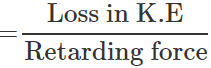As both the kinetic energy and retarding force are same, so both the lorry and the car would come to rest in the same distance.
Q.38 A truck and a car are moving with the same kinetic energy on a straight road. Their engines are simultaneously switched off. Which one will stop at a lesser distance?
Answer:
By work-energy theorem, Loss in K.E. of the vehicle =Work done against the force of friction x distance or K.E. =f×s=μR×s=μmg s or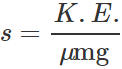For constant K.E., s∝ 1/m As the truck has more mass than the car, so it will stop in a lesser distance than the car.
Q.39 A rocket explodes in mid air. How does this affect (a) its total momentum and (b) its total kinetic energy?
Answer:
(a) Because no external force acts on the rocket, its total momentum remains unchanged. (b) When the rocket explodes, its fragments receive additional kinetic energy from the explosion. The chemical energy of the fuel changes into kinetic energy. As a result of this, the total kinetic energy gets increased.
Q.40 The velocity of an aeroplane is doubled. (a) What will happen to its momentum? Will the momentum remain conserved? (b) What will happen to its K.E.? Will the energy remain conserved?

Answer: (a) when the velocity of the aeroplane is doubled, its momentum also gets doubled. However, the combined momentum of aeroplane + air is conserved. As the momentum of the aeroplane increases, the momentum of air also increases by an equal amount in the opposite direction. (b) The kinetic energy becomes four times. The additional energy is obtained by burning of fuel. However, the total energy is still conserved.
Q.41 A uniform rectangular parallelopiped of mass m having sides l, 2l and 4l is placed in turn on each of its three sides on a horizontal surface. What is the potential energy of the parallelepiped in each of these positions? Which position will be the most stable?
Answer:
When the parallelepiped is placed on its small, middle and large side, its center of gravity will lie at heights 2l,land l/2 respectively above the horizontal surface. If U1,Uand U3are the potential energies in the three Cases, then U1=mg×2l=2mgl U2=mg×l=mgl U3=mg×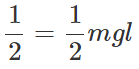When the parallelepiped rests on its large side, its potential energy is minimum. So this position is the position of stable equilibrium.
Q.42 What happens to the potential energy when (a) two protons are brought close together (b) one proton and one electron are brought close together?
Answer:
(a) The potential energy increases when two protons are brought closer because work has to be done against the force of repulsion between them. (b) The potential energy decreases when a proton and an electron are brought closer because work is done by the force of attraction between them (origin of the force being the system itself).
Q.43 A particle is moving in a circular path of a given radius with number of rotations per second (i) constant, (ii) increasing, (iii) decreasing. What happens to work done in the three cases?

Answer: Work is equal to the change in kinetic energy of the system, (i) In case speed is constant, kinetic energy does not change, hence work done is zero, (ii) when speed increases, K.E. increases, work is done by the exerting force, (iii) when speed decreases, K.E. decreases, work is done by the body on the force.
Q.44 "Chemical, gravitational and nuclear energies are nothing but potential energies for different types of forces in nature." Explain the statement clearly with examples.

Answer: A system has potential energy, when various objects are held at certain distance against some force, by virtue of their position or configuration. Chemical potential energy results from the chemical bonding of atoms, gravitational potential energy results when objects are held at some distance against gravitational force while nuclear energy arises from the nuclear force between the nucleons.
Q.45  In a thermal power station, coal is used for the generation of electricity. Mention how energy changes from one form to another before it is transformed into electrical energy?

Answer: The heat energy produced due to combustion of coal converts water into steam. The heat energy of steam is converted into mechanical energy when it turns blades of a turbine. The mechanical energy so obtained is converted into electrical energy by the generators.
Q.46 Explain how fast moving neutrons can be quickly slowed down by passing through, water or heavy water.
Answer:
Water and heavy water contain protons having approximately the same mass as that of a neutron. When fast moving neutrons collide with protons, the neutrons attain the low velocities of protons while the protons begin to move with the high velocities of neutrons.
Q.47 Two ball bearings of mass m each moving in the opposite directions with equal speed υ collide head on with each other. Predict the outcome of the collision, assuming it to be perfectly elastic.

Answer: Here m1=m2=m,u1=υ, u2=−υ ∴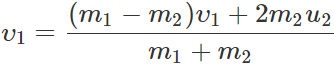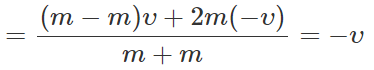and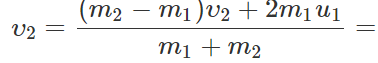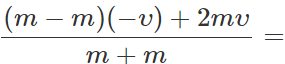υHence after collision, the two ball bearings will move with same speeds but their directions of motion are reversed.
Q.48 A body of mass m moving with speed υcollides elastically head-on with another body of mass m initially at rest. Show that the moving body will come to a stop as a result of this collision.

Answer: By conservation of momentum, m×υ+m×0=mυ1+mυ2 or υ=υ12 or            υ−υ12 ?(1) By conservation of kinetic energy,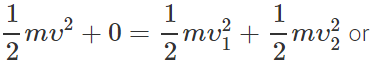υ2=υ2122 or (υ+υ1)(υ−υ1)=υ2   ?(2) Dividing (2) by (1), we get υ+υ12 ?(3) Solving (1) and (3), υ1=0 and υ2=υ As a result of the collision, the moving body comes to a stop and the stationary body begins to move with the speed of the first body.
Q.49 A uniform chain of length L and mass M is lying on a smooth table and one third of its length is hanging vertically down over the edge of the table. If g is acceleration due to gravity, calculate work required to pull the hanging part on the chain.
Answer: Weight of length L of the chain =Mg Weight of length L/3 of the chain = 1/3 Mg As the center of gravity of the hanging part lies at its midpoint i.e. at a distance equal to L/6 below the edge of the table, so the work required to pull the hanging part on the table is W =Force × distance =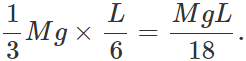Q.50 Why a metal ball rebounds better than a rubber ball?
Answer:
When a rubber ball hits a massive object, say, earth, the ball is distorted. A large amount of heat is generated in the ball by the rubbing of the rubber molecules against each other. This effect is essentially absent in a hard material. So, a metal ball would often lose less energy upon collision than would a rubber ball.
Q.51 Explain, throwing mud on a wall is an example of perfectly inelastic collision.

Answer: When mud is thrown on a wall, it sticks to the wall. The kinetic energy of the mud is reduced to zero and there is non-conservation of kinetic energy. Hence it is a case of perfectly inelastic collision.
Q.52 Nuclear fission and fusion reactions are examples of conversion of mass into energy. Can we say that strictly speaking, mass is converted into energy even in an exothermic chemical reaction?

Answer: Yes, the generation of heat in exothermic reaction involves the conversion of mass into energy. However, the amount of mass involved is much less than that in fission or fusion.
Q.53 The energy released in fusion reaction of light nuclei is much less than the energy released in a fission reaction of a heavy nucleus. Why is then a hydrogen bomb (based on nuclear fusion) far more powerful than an atomic bomb (based on nuclear fission)?

Answer: A hydrogen bomb (based on nuclear fusion) is more powerful than atom bomb (based on nuclear fission), because in case of fusion, the energy released per unit mass of the fuel is much larger than that in fission.
Q.54 By convention, the forces which fall off to zero at large distances, the potential energy at infinity is taken to be zero. With this choice, is the potential energy positive for (a) electron-positron bound state (b) planet- satellite system and (c) electron-electron system?

Answer: (a) Negative, because of attraction between electron and positron. (b) Negative, because of attraction between planet and satellite. (c) Positive, because of repulsion between two electrons.

Offer running on EduRev: Apply code STAYHOME200 to get INR 200 off on our premium plan EduRev Infinity!

## Physics For JEE

188 videos|346 docs|307 tests

,

,

,

,

,

,

,

,

,

,

,

,

,

,

,

,

,

,

,

,

,

,

,

,

;